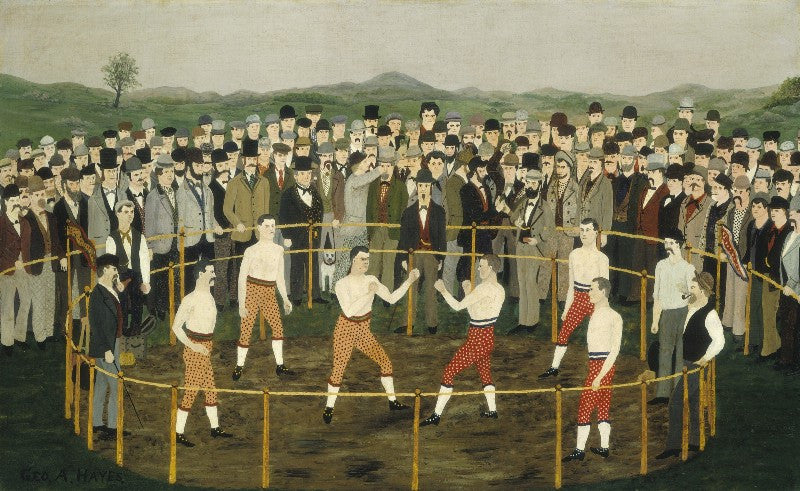### Boxing Match

Dan and Dave are packing up orders at the Art of Play warehouse. Dan presents Dave with three boxes and asks him to print out shipping labels. Dave asks, “How much do they weigh?”

Dan smiles and replies, “The sum of their weights is your lucky number.” Dave sighs.

“I need more specifics.” Dave says.

“OK,” says Dan, “the product of their weights equals seventy-two.” Dave thinks for a moment.

“That’s still not enough information.” he says as he looks around for the scale.

“Hold on,” says Dan, “I have one more clue: the heaviest box contains my favorite thing in the whole store.”

Dave counts on his fingers for a few moments, then types up the shipping labels and places the correct postage on each package.

What are the individual weights of the three boxes?

Solution: First you need to figure out the various sets of three numbers that multiply to 72. When you look at the list of products and then compare their sums, you notice that two sets of numbers equal 14. This must be the lucky number because we still need more information to solve and any other total is a unique set of three. The last clue tells us there is a "heaviest box" which means the set can not be 6-6-2 and must be 8-3-3.

Be among the first to solve our next brain-teaser and win a cool prize. Follow us on Instagram and watch for new puzzles with contest details.

Write a commentBear Smith

8,3,3 gramsTom

3 4 6Benjamin Loo

The packages weigh 3, 4 & 6 kg respectively.John Alec Mari Cosico

So the first question was how much do they weigh.

X + Y + Z = W (W as Lucky Number)

Here are some of the possibilities to have a product to 72 then possibilities of sum of the magic number

1+1+72=74
1+2+36=39
1+4+18=25
1+8+9=18
2+4+9=15
3+3+8=14
3+4+6=13

So of course you can’t count for a few moments numbers above 10, so the possibilities that sum 25 up are not counted. So the possible answers for the magic numbers are 18, 15, 14, and 13 as shown above. So, i just assumed that 13 is the lucky number since 13 = Kings.

As for the favorite thing, I just hope I’m lucky that it weighs 6 (lbs,kg,g, etc) (on what unit it uses)

3, 4 and 6.

Hoping to win! :DCody Shi

The numbers are 3,4, and 6Bobby

2, 4, 9Pranav

8, 3, 3Bear Smith

2 grams 4 grams and 9 grams.
Because a pack of 52 cards weighs about 3.3 grams and the highest number is nine which is divisible by 3 decks of cards.Jhaz Pierce

The individual weights of the three boxes are 8, 3, and 3
With a lucky number of 14Alain Lacourse

I need to change my solution. There is an issue with my previous one. The solution cannot be 2-3-12 because it is the only one that sums up to 17, and Therefore, a third clue would not be needed.

The answer is rather 3-3-8. The product is still 72. The sum is 14, and there is another combination that sums to 14: 2-6-6. Therefore a third clue is indeed required to solve the puzzle. However, 2-6-6 has two biggest boxes, not one like the grammar of the sentences indicate. Moreover, the Hollingworths deck would be Dan’s favorite thing since this deck is 8 cards thinner than a standard bicycle deck.

So the solution is 2-6-6.Marcello

3,4,6Diego Gonzalez

3, 3, and 8 weight units.Mark Saren

The answer is 8, 3, and 3. There are only to options to the weights of the packages based on the first 2 hints, 6, 6, 2; and 8, 3, 3 because they are the combinations that has a product of 72, and the same sum (the lucky number). The last hint, “The heaviest box…”, rules out 6, 6, 2, and that leaves 8, 3, 3 as the answer.Thinh

3,3,8Aaron Curtis

3 4 6Connor McGeorge

3,3, and 8Dave knew his lucky number but there were multiple answers for that so he needed more information to narrow down the choice, so Dan told him that the product of them is 72 but he still needed more information because there is more than one option that have the sum of his lucky and the only two options that have the same sum are 3,3,8 and 2,6,6 so when Dan told him that the heaviest one has his favorite thing he knew that it can’t be 2,6,6 because in this option there is no heaviest box and that means that the right answer is 3,3,8.Chris Bell

It should be 3, 3, and 8. The first two questions pose that three numbers, when added, equals some number, with the product of those SAME numbers equating to 72. As many combinations as there are, there are only two combinations that produce the same number as a lucky number, which is why he was confused. You then adding that there was a heaviest one (implying a single heaviest box) then gives a hint that the duplicate number is not the biggest number.larry meng

The weights are
3
3
8larry meng

The weights are
3
3
8Scott Greene

Or three four sexScott Greene

3 4 6Jarrod F.

3, 4, and 6Bailey Anderes

24 units of weightguillermo

The first one 3,the second one 3 and the last one 8